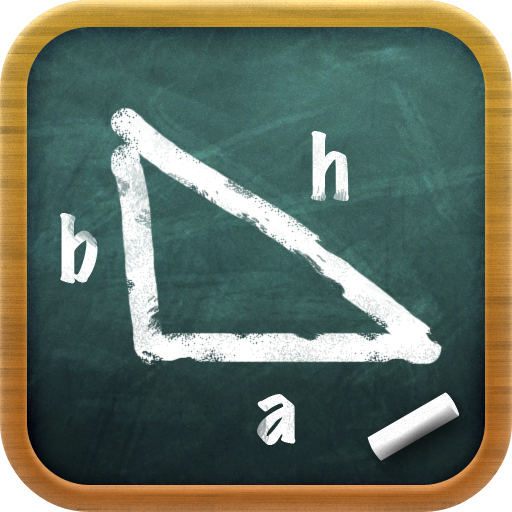## Welcome

New:Awarded "Best" at iPhoneAppsPlus: http://www.iphoneappsplus.com/education/imath/index.htm

iMath, the best easy-to-use math learning appWith iMath you can easily:
- Calculate quadratic equations
- Calculate pythagoras theorem
- Calculate logarithms
- Send a custom math problem to be solved
- Learn the formulas so you can solve it by yourself!

Get iMath on AppStore today!

Email us your suggestions so we can make it even better